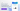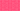Pricing# What is a basis point?

Written by

When looking at changes to yield spreads or interest rates, the smallest possible unit of measurement is a basis point. Keep reading to find out how basis points are used in finance, and what their benefits are.

## Introduction to basis points

Also known as ‘bips’ or bps, basis points are used in finance as units of measurement. A single basis point is the equivalent to 1/100th of a percent:

1 bps = 0.01% = 0.0001

They provide a high level of accuracy when tracking percentage changes for a variety of financial rates or values. Typical examples include interest rates and bond yields. You can express basis points in fractions for even greater accuracy. For example, 1.5 bps would be the equivalent to 0.015%.

You’ll often see or hear references to basis points in financial reporting. If an investor states that the yield on a Treasury bond fell by 20 basis points, this would mean that it dropped by 0.2%. Using this term helps prevent confusion when it comes to discussing differences in rates, which we’ll explore below.

## How are basis points used?

Basis points are primarily used when measuring small changes to interest rates or yields, but they can also be applied to changes in an asset’s value. For example, when stock values change by percentages lower than 1%, basis points are used to describe these changes. Here’s a list of some of the financial instruments that can be measured with basis points:

• Corporate bonds

• Treasury bonds

• Interest rates

• Credit derivatives

• Options and futures

• Debt securities

• Equity securities

## What are the benefits of bps?

When investors track changes in value, they’re often dealing with very small percentages. Basis points offer a highly accurate way to describe these small changes in value. A secondary benefit is that using bps helps prevent ambiguity when reporting the differences between relative and absolute interest rates.

For example, imagine you are discussing an interest rate that has increased from 10% to 11%. Under the absolute method, you would say that there was a 1% increase. However, under the relative method this might be a different figure entirely. To avoid any confusion when describing multiple types of percentages, you can simply use basis points instead. In this case, you’d say that there was an increase of 100 basis points, equalling 1%. Because 1 bps is always equivalent to 0.01%, there is no room for confusion. It’s a universal measurement that can be applied to many different financial instruments.

A third benefit to using basis points is that you can track changes to the stock index in a clear-cut way. Analysts often find it easier to discuss percentage changes in asset value using the points-based system of bps rather than dealing in percentages, which can get muddled up with other types of rate increases.

## Basis point calculator

Converting basis points to percent and vice versa is simple, using the following easy calculation:

• Percent to basis points: multiply percentage by 100

• Basis points to percent: divide points by 100

Using this calculation, 10,000 bps equals 100%.

Here’s a simple chart showing the progression of basis points and percentage:

 Basis Points Percentage 1 0.01% 10 0.1% 50 0.5% 75 0.75% 100 1% 1000 10%

Using this basis point calculator chart, you can quickly work out what the bps would be of any change to rates. For example, the difference between bond interest rates of 4.75% and 4.25% would be 0.5%, or 50 bps. Another example would be if Company A’s stock increased by 220 bps in a single day, it grew by 2.2%.

Overall, basis points provide a simple, user-friendly way to express changes to yields and interest rates. Yields are subject to constant fluctuations, which makes this type of measurement extremely useful.

## We can help

GoCardless helps you automate payment collection, cutting down on the amount of admin your team needs to deal with when chasing invoices. Find out how GoCardless can help you with ad hoc payments or recurring payments.